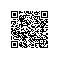# ruby 的while

while 是一个非常强大的语句，很大程度上可以替换其他判断和循环。

1、while 代替if

 1 2 3 while 5 > 3   puts "ok" end

2、while 代替 for 循环

 1 2 3 4 5 6 7 sum = 0 i = 1 while i <= 5   sum += i   i += 1 end puts sum

3、while代替until

 1 2 3 4 5 6 7 sum = 0 i = 1 while !(sum >= 50)   sum += i   i += 1 end puts sum使用钉钉扫一扫加入圈子
+ 订阅